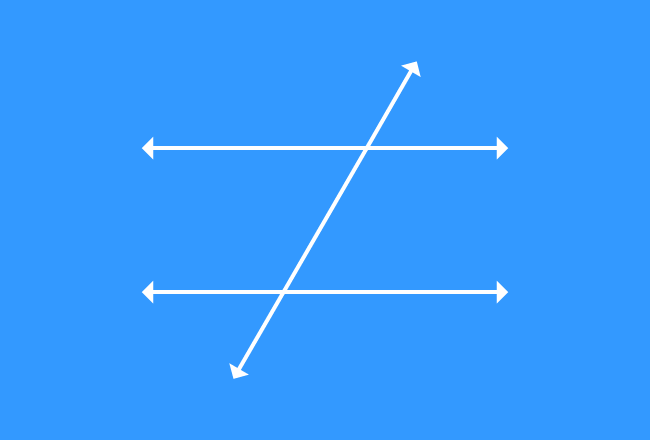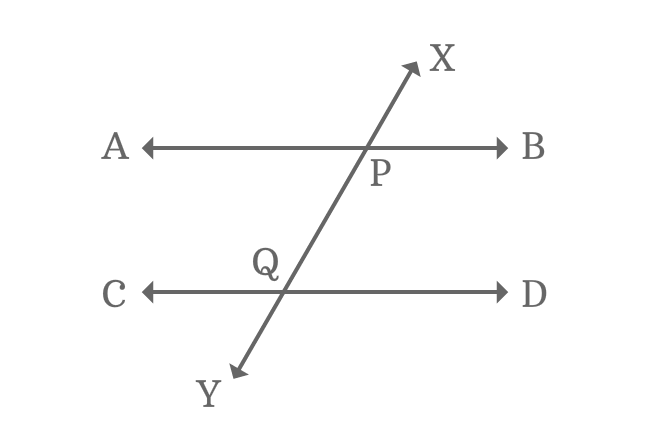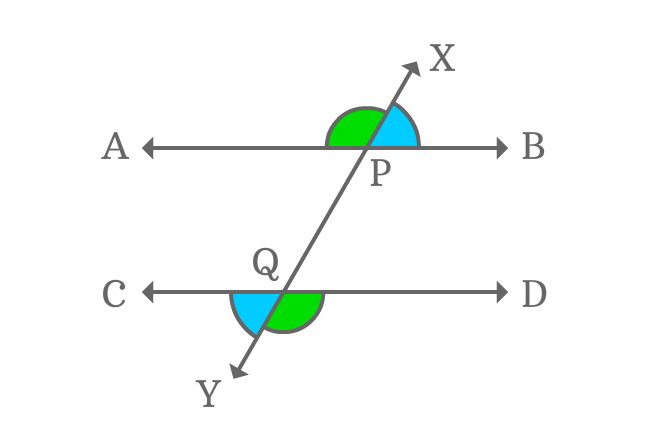# Alternate Exterior angles formed by Parallel lines and their TransversalWhen two or more parallel lines are intersected by their transversal, the exterior angles which appear oppositely are called alternate exterior angles or exterior alternate angles of parallel lines and their transversal.

Two pairs of exterior angles are formed on opposite side at the intersection of two parallel lines and transversal. Therefore, the two pairs of exterior angles are called alternate exterior angles and also called as exterior alternate angles.

## Alternate Exterior angles$\angle APX$, $\angle XPB$, $\angle DQY$ and $\angle YQC$ are four exterior angles, formed by the intersection of parallel lines ($\overleftrightarrow{AB}$ and $\overleftrightarrow{CD}$) and their transversal $\overleftrightarrow{XY}$.

$(1) \,\,\,\,\,\,$ $\angle APX$ and $\angle DQY$

$(2) \,\,\,\,\,\,$ $\angle XPB$ and $\angle YQC$

Each pair of above angles are exterior angles and also appear on opposite side of the intersection of parallel lines and their transversal. So, they are known as exterior alternate angles.

### PropertyEach pair of exterior alternate angles are equal due to the parallelism property of the parallel lines.

$(1) \,\,\,\,\,\,$ $\angle APX = \angle DQY$

$(2) \,\,\,\,\,\,$ $\angle XPB = \angle YQC$

Therefore, it is proved that every pair of alternate exterior angles are equal when two parallel lines are cut by their transversal line.

Latest Math Topics
Jun 26, 2023
Jun 23, 2023

Latest Math Problems
Jul 01, 2023
Jun 25, 2023
###### Math Questions

The math problems with solutions to learn how to solve a problem.

Learn solutions

Practice now

###### Math Videos

The math videos tutorials with visual graphics to learn every concept.

Watch now

###### Subscribe us

Get the latest math updates from the Math Doubts by subscribing us.